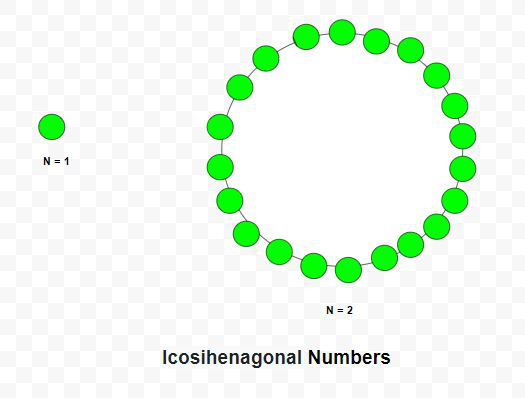# Icosihenagonal Number

Given a number N, the task is to find Nth Icosihenagonal number.

An Icosihenagonal number is class of figurate number. It has 21 – sided polygon called Icosihenagon. The n-th Icosihenagonal number counts the 21 number of dots and all others dots are surrounding with a common sharing corner and make a pattern. The first few Icosihenagonal numbers are 1, 21, 60, 118, 195, 291, 406 …

Examples:

Input: N = 2
Output: 21
Explanation:
The second Icosihenagonal number is 21

Input: N = 6
Output: 291

## Recommended: Please try your approach on {IDE} first, before moving on to the solution.Approach: In mathematics, the Nth Icosihenagonal number is given by the formula:

``````

Below is the implementation of the above approach:

## C++

 `// C++ program to find nth ` `// Icosihenagonal number ` ` `  `#include ` `using` `namespace` `std; ` ` `  `// Function to find ` `// Icosihenagonal number ` `int` `Icosihenagonal_num(``int` `n) ` `{ ` `    ``// Formula to calculate nth ` `    ``// Icosihenagonal number ` `    ``return` `(19 * n * n - 17 * n) / 2; ` `} ` ` `  `// Driver Code ` `int` `main() ` `{ ` `    ``int` `n = 3; ` `    ``cout << Icosihenagonal_num(n) << endl; ` ` `  `    ``n = 10; ` `    ``cout << Icosihenagonal_num(n) << endl; ` ` `  `    ``return` `0; ` `} `

## Java

 `// Java program to find nth ` `// Icosihenagonal number ` `class` `GFG{ ` ` `  `// Function to find ` `// Icosihenagonal number ` `static` `int` `Icosihenagonal_num(``int` `n) ` `{ ` `    ``// Formula to calculate nth ` `    ``// Icosihenagonal number ` `    ``return` `(``19` `* n * n - ``17` `* n) / ``2``; ` `} ` ` `  `// Driver Code ` `public` `static` `void` `main(String[] args) ` `{ ` `    ``int` `n = ``3``; ` `    ``System.out.print(Icosihenagonal_num(n) + ``"\n"``); ` ` `  `    ``n = ``10``; ` `    ``System.out.print(Icosihenagonal_num(n) + ``"\n"``); ` `} ` `} ` ` `  `// This code is contributed by Rajput-Ji `

## Python3

 `# Python3 program to find nth  ` `# icosihenagonal number  ` ` `  `# Function to find  ` `# icosihenagonal number  ` `def` `Icosihenagonal_num(n): ` `     `  `    ``# Formula to calculate nth  ` `    ``# icosihenagonal number ` `    ``return` `(``19` `*` `n ``*` `n ``-` `17` `*` `n) ``/` `2` `     `  `# Driver Code ` `n ``=` `3` `print``(``int``(Icosihenagonal_num(n))) ` ` `  `n ``=` `10` `print``(``int``(Icosihenagonal_num(n))) ` ` `  `# This code is contributed by divyeshrabadiya07 `

## C#

 `// C# program to find nth ` `// Icosihenagonal number ` `using` `System; ` ` `  `class` `GFG{ ` ` `  `// Function to find ` `// Icosihenagonal number ` `static` `int` `Icosihenagonal_num(``int` `n) ` `{ ` `    ``// Formula to calculate nth ` `    ``// Icosihenagonal number ` `    ``return` `(19 * n * n - 17 * n) / 2; ` `} ` ` `  `// Driver Code ` `public` `static` `void` `Main() ` `{ ` `    ``int` `n = 3; ` `    ``Console.Write(Icosihenagonal_num(n) + ``"\n"``); ` ` `  `    ``n = 10; ` `    ``Console.Write(Icosihenagonal_num(n) + ``"\n"``); ` `} ` `} ` ` `  `// This code is contributed by Code_Mech `

Output:

```60
865
```

Don’t stop now and take your learning to the next level. Learn all the important concepts of Data Structures and Algorithms with the help of the most trusted course: DSA Self Paced. Become industry ready at a student-friendly price.

My Personal Notes arrow_drop_upCheck out this Author's contributed articles.

If you like GeeksforGeeks and would like to contribute, you can also write an article using contribute.geeksforgeeks.org or mail your article to contribute@geeksforgeeks.org. See your article appearing on the GeeksforGeeks main page and help other Geeks.

Please Improve this article if you find anything incorrect by clicking on the "Improve Article" button below.

Article Tags :
Practice Tags :

Be the First to upvote.

Please write to us at contribute@geeksforgeeks.org to report any issue with the above content.